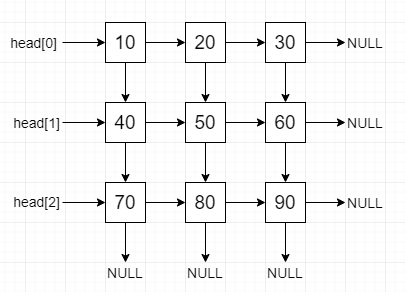# Construct a linked list from 2D matrix (Iterative Approach) in C++

Suppose we have one matrix, we have to convert it to 2d linked list using iterative approach. The list will have the right and down pointer.

So, if the input is like

 10 20 30 40 50 60 70 80 90

then the output will beTo solve this, we will follow these steps −

• Define an array head_arr of size: m.

• for initialize i := 0, when i < m, update (increase i by 1), do −

• for initialize j := 0, when j < n, update (increase j by 1), do −

• p := new tree node with value mat[i, j]

• if real_head is null, then −

• if head_arr[i] is null, then −

• Otherwise

• right of right_ptr := p

• right_ptr := p

• for initialize i := 0, when i < m - 1, update (increase i by 1), do −

• while (p and q both are not null), do −

• down of p := q

• p := right of p

• q := right of q

## Example

Let us see the following implementation to get better understanding −

Live Demo

#include <bits/stdc++.h>
using namespace std;
class TreeNode {
public:
int data;
TreeNode *right, *down;
TreeNode(int d){
data = d;
right = down = NULL;
}
};
while (down_ptr) {
right_ptr = down_ptr;
while (right_ptr) {
cout << right_ptr->data << " ";
right_ptr = right_ptr->right;
}
cout << endl;
down_ptr = down_ptr->down;
}
}
TreeNode* make_2d_list(int mat[], int m, int n) {
TreeNode *right_ptr, *p;
for (int i = 0; i < m; i++) {
for (int j = 0; j < n; j++) {
p = new TreeNode(mat[i][j]);
else
right_ptr->right = p;
right_ptr = p;
}
}
for (int i = 0; i < m - 1; i++) {
while (p && q) {
p->down = q;
p = p->right;
q = q->right;
}
}
}
int main() {
int m = 3, n = 3;
int mat[] = {
{ 10, 20, 30 },
{ 40, 50, 60 },
{ 70, 80, 90 } };
TreeNode* head = make_2d_list(mat, m, n);
}

## Input

{ { 10, 20, 30 },
{ 40, 50, 60 },
{ 70, 80, 90 } }

## Output

10 20 30
40 50 60
70 80 90